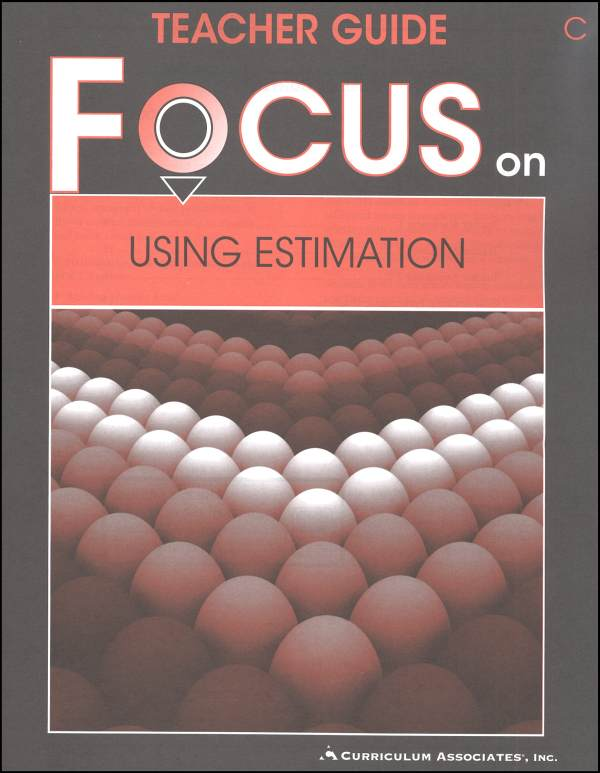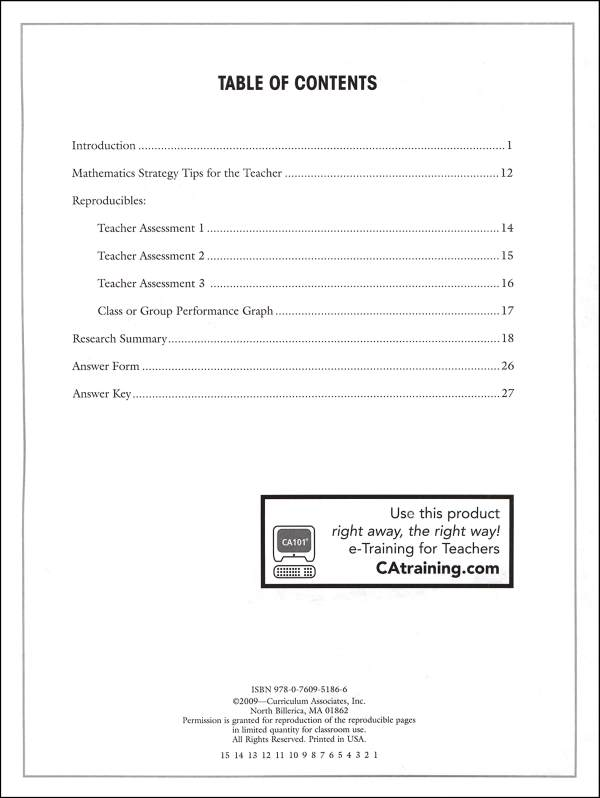••# Using Estimation Teacher Guide C

# 044045

Our Price: \$0.77
Retail: \$6.95
Save: 88.92% (\$6.18)
4 In Stock. Item will be unavailable when sold out.
Qty:
Qty:

 Item #: 044045 9780760951866 3

Category Description for Focus On... Mathematics:

This extensive series does exactly what the title suggests – each booklet allows students to focus on specific math skills. Six different math areas are available for each grade to reinforce math skills. These books are simple to implement and students can use them for independent practice.

Every student workbook follows the same format. The first section introduces the program. The table of contents is next, and is followed by Learn About. This section is two pages in length and presents the concepts to be practiced in this particular book along with basic instruction and samples. A two-page lesson preview gives practice exercises and explains why answers chosen might be correct or incorrect. There are 20 lessons in each book, each two pages in length with only 5 problems, to be used for independent practice. A tracking chart, self-assessments, and an answer form (fill-in-the-dot) are found at the back of the book.

There is one teacher guide to accompany each of the student books. You will find suggested instructions, strategies and tips, reproducibles for student assessment, a summary of the research behind the series, and completed answer forms along with the actual answers and possible answers to questions in the student book. There is also a suggested schedule for when and how to use this series.

Building Number Sense develops counting skills in grades 1-3 and introduces the concept of ordinal numbers. Grades 4-8 begin with the concept of fractions and build using decimals, percents, exponents, and square roots. Grade 8 introduces students to prime and composite numbers and practice with order of operations when solving problems.

Determining Probability and Averages introduces younger children to the concepts of ‘more likely,’ ‘less likely,’ and ‘equally likely.’ Students in grades 2-8 learn how to average. In grades 7 and 8 they are introduced to mean, median, mode and range.

Interpreting Graphs & Charts introduces students to various types of charts, and they learn to read and interpret the information as they grow in complexity from level to level.

Using Algebra sounds like a pretty complex topic for young children, but not the way it is presented in this series. In earlier grades students learn to use simple number sentences with missing information, and try to identify the missing information - these are fill-in-the-blank for grades 1 and 2. Beginning at grade 3 letter variables are introduced, and as they progress through the grades they will write and solve equations, expressions, and inequalities.

Using Estimation begins in grades 1 and 2 by estimating with 2-digit numbers. A progression through the levels will lead to estimating differences, products, and quotients. Older students will estimate with mixed numbers, percents, and money.

Using Geometry begins by recognizing common shapes, distance around a figure (perimeter), and equal halves. Grade 3 introduces congruent figures, grade 4 introduces area, volume in grade 5, graphing a slide in grade 7, and parallel lines and transversals in grade 8.

This series would be great for extra practice, skill reinforcement, or test preparation. The A through G levels correspond sequentially to grades 1-8 and provide that little bit extra you might be missing. ~ Donna

Primary Subject
Mathematics
3
ISBN
9780760951866
Format
Softcover Book
Brand Name
Curriculum Associates
Weight
0.15 (lbs.)
Dimensions
11.0" x 9.0" x 0.06"